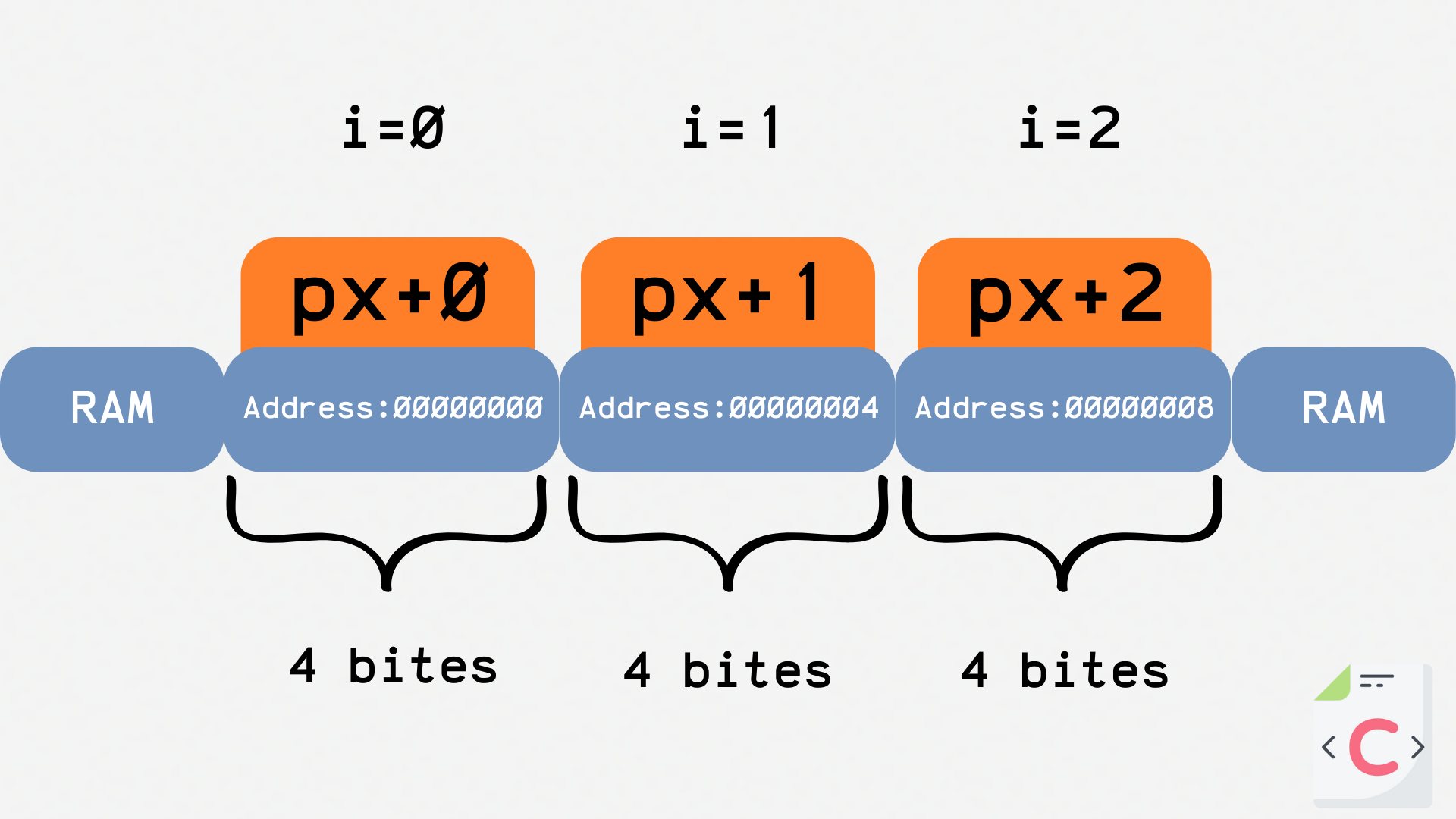Course Content

C Basics

##Functions, Arrays and Two Pointers

### Function with pointer

Let's try using a regular function to change the value of our data. For example, you need a function that would convert kilo-ohms to ohms (1 kOhm = 1000 Ohm).Main.cWe were unable to change the value of the `r` variable. And all because not the value of the `r` variable itself is substituted into the function, but its copy.

In order for our program to make sense, we must substitute the address of the `r` variable into the function, therefore, the `Ohm` function must accept not `double`, but `double*`.Main.cNote that we are accessing the `r` variable twice. After using the `Ohm` function, the value of `r` has changed. And all because the function got not a copy but the original variable `r` address, and the function simply changed the value at the specified address.

Also, a function can return a pointer to an object that it itself has created:Main.c### Are arrays just pointers?

What do you think will happen if a number is added to the address?Main.cIf we add a number (`pX + 1`) to the address, we will get the address of the next memory cell!

Let's write a loop that will help us see the "sequence" of RAM:Main.cWe have looked ahead 3 steps. It turns out that among the received addresses, there is a clear hierarchy.Since the `int` type takes up 4 bytes, we move forward 4 bytes per step. Very similar to an array!

It turns out that an array is just a constant address (which is hidden under the array's name) and allocated memory, and the index of the elements is their offset relative to the address of the first element!

We can prove this with the following program:Main.cAs you can see, we are not going straight through the array. We only use its address (the address of its first element).

Everything was clear?

Section 6. Chapter 5